# Variational Autoencoder with Tensorflow 2.8 – II – an Autoencoder with binary-crossentropy loss

In the last post of this series

Variational Autoencoder with Tensorflow 2.8 – I – some basics

I have briefly discussed the basic elements of a Variational Autoencoder [VAE]. Among other things we identified an Encoder, which transforms sample data into z-points in a low dimensional „latent space“, and a Decoder which reconstructs objects in the original variable space from z-points.

In the present post I want to demonstrate that a simple Autoencoder [AE] works as expected with Tensorflow 2.8 [TF2]. In contrast to certain versions of Variational Autoencoders which we shall test in the next post. For our AE we use the „binary cross-entropy“ as a suitable loss to compare reconstructed MNIST images with the original ones.

# A simple AE

I use the functional Keras API to set up the Autoencoder. Later on we shall encapsulate everything in a class, but lets keep things simple for the time being. We design both the Encoder and the Decoder as CNNs and use properly configured Conv2D and Conv2DTranspose layers as their basic elements.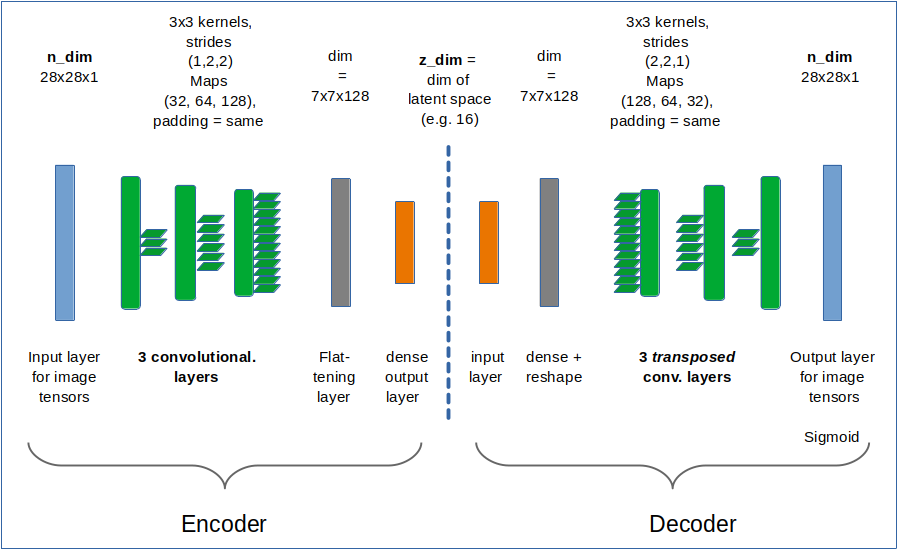Required Imports

```# tensorflow and keras
import tensorflow as tf
from tensorflow import keras as K
from tensorflow.keras import backend as B
from tensorflow.keras.models import Model
from tensorflow.keras import regularizers
from tensorflow.keras import metrics
from tensorflow.keras.layers import Input, Conv2D, Flatten, Dense, Conv2DTranspose, Reshape, Lambda, \
Activation, BatchNormalization, ReLU, LeakyReLU, ELU, Dropout, \

from tensorflow.keras.utils import to_categorical
from tensorflow.keras.optimizers import schedules
```

z_dim and the Encoder defined with the functional API
We set the dimension of the „latent space“ to z-dim = 16.
This allows for a very good reconstruction of images in the MNIST case.

```z_dim = 16
encoder_input = Input(shape=(28,28,1)) # Input images pixel values are scaled by 1./255.
x = encoder_input
x = Conv2D(filters = 32, kernel_size = 3, strides = 1, padding='same')(x)
x = LeakyReLU()(x)
x = Conv2D(filters = 64, kernel_size = 3, strides = 2, padding='same')(x)
x = LeakyReLU()(x)
x = Conv2D(filters = 128, kernel_size = 3, strides = 2, padding='same')(x)
x = LeakyReLU()(x)
shape_before_flattening = B.int_shape(x)[1:]  # B: Keras backend
x = Flatten()(x)
encoder_output = Dense(self.z_dim, name='encoder_output')(x)
encoder = Model([encoder_input], [encoder_output], name="encoder")
```

This is almost an overkill for something as simple as MNIST data. The Decoder reverses the operations. To do so it uses Conv2DTranspose layers.

The Decoder

```dec_inp_z      = Input(shape=(z_dim))
x = Dense(np.prod(shape_before_flattening))(dec_inp_z)
x = Reshape(shape_before_flattening)(x)
x = Conv2DTranspose(filters=64, kernel_size=3, strides=2, padding='same')(x)
x = LeakyReLU()(x)
x = Conv2DTranspose(filters=32, kernel_size=3, strides=2, padding='same')(x)
x = LeakyReLU()(x)
x = Conv2DTranspose(filters=1,  kernel_size=3, strides=1, padding='same')(x)
x = LeakyReLU()(x)
# Output into a region of 0 to 1 - requires a one hot encoding of classes
# and scaling of pixel values of the input samples to [0, 1]
x = Activation('sigmoid')(x)
decoder_output = x
decoder = Model([dec_inp_z], [decoder_output], name="decoder")
```

The full AE-model

```ae_input  = encoder_input
ae_output = decoder(encoder_output)
AE = Model(ae_input, ae_output, name="AE")
```

That was easy! Now we compile:

```AE.compile(optimizer=Adam(learning_rate = 0.0005), loss=['binary_crossentropy'])
```

I use the „binary_crossentropy“ loss function to evaluate and punish differences between the reconstructed image and the original. Note that we need to scale pixel values of input images (as MNIST images) down to a value range between [0, 1] due to using a sigmoid activation function at the output layer of the Decoder.
Using „binary cross-entropy“ leads to a better and faster convergence of the training process:

# Training and results of the Autoencoder for MNIST samples

Eventually, we can train our model:

```n_epochs = 40
batch_size = 128
AE.fit( x=x_train, y=x_train, shuffle=True,
epochs = n_epochs, batch_size=batch_size)
```

After 40 training steps we can visualize the resulting data clusters in z-space. To get a 2-dimensional plot out of 16-dimensional data requires the use of t-SNE. For 15.000 training data (out of 60.000) we get:

Our Encoder CNN was able to separate the different classes quite well by extraction basic features of the handwritten digits by its Conv2D layers. The test which the AE never had seen before are well separated too:

Note that the different position of the clusters on the 2-dim plots are due to transformations and arrangements of t-SNE and have no deeper meaning.

For z_dim = 16 it is almost perfect: Below you see a sequence of rows presenting original images and their reconstructed counterparts for selected MNIST test data, which the AE had not seen before: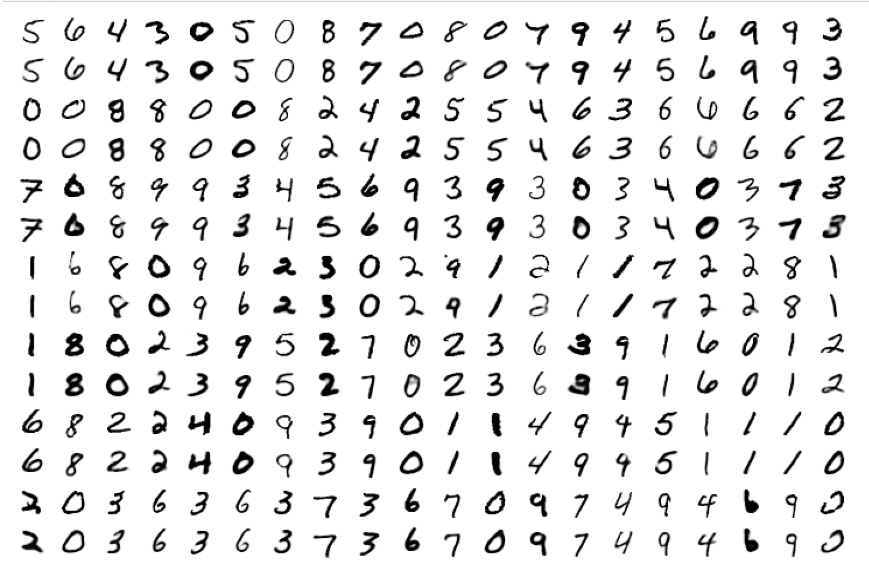# The special case of „z_dim = 2“

When we set z_dim = 2 the reconstruction quality of course suffers: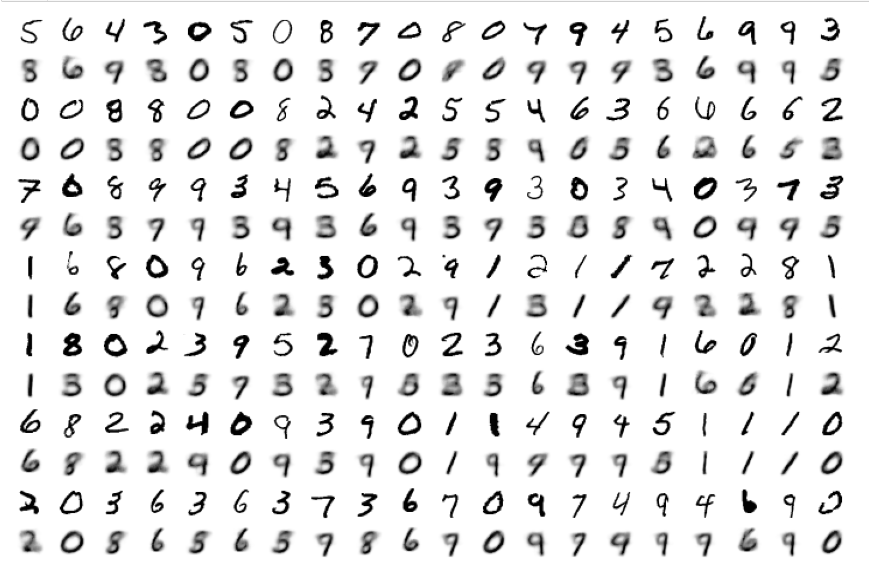Regarding class related clusters we can directly plot the data distributions in the z-space: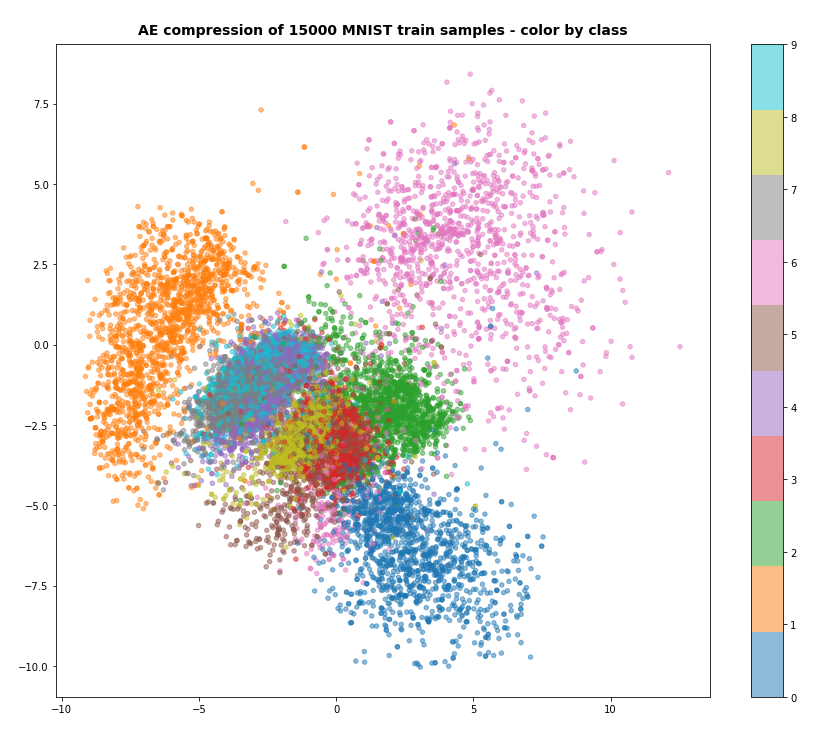We see a relatively vast spread of data points in clusters for „6“s and „0“s. We also recognize the typical problem zones for „3“s, „5“s, „8“s on one side and for „4“s, „7“s and „9“s on the other side. They are difficult to distinguish with only 2 dimensions.

# Conclusion

Autoencoders are simple to set up and work as expected together with Keras and Tensorflow 2.8.

In the next post

Variational Autoencoder with Tensorflow 2.8 – III – problems with the KL loss and eager execution

we shall, however, see that VAEs and their KL loss may lead to severe problems.

Ceterum censeo: The worst living fascist and war criminal today, who must be isolated, denazified and imprisoned, is the Putler.

# KMeans as a classifier for the WIFI and MNIST datasets – I – Cluster analysis of the WIFI example

In the November and December 2021 editions of the German „Linux Magazin“ R. Pleger discussed a simple but nevertheless interesting example for the application of a cluster algorithm. His test case was based on a dataset of the UCI Irvine. This dataset contains 2000 samples with (fictitious?) data describing WIFI signals which stemmed from seven WLAN spots around a building. The signal strength of each source was measured at varying positions in four different rooms. I call the whole setup the „WIFI example“ below.

One objective of the articles in the Linux Magazin was to demonstrate how simple it is today to apply basic Machine Learning methods. In a first step the author used a ML classifier algorithm to determine the location (i.e. the room) of a measuring instrument just from the strengths of the different WIFI signals. This task can be solved by a variety of algorithms – e.g. by a Decision Tree, SVM/SVC or a simple Multilayer Perceptron. The author used Sklearn’s RandomForestTree. This method is a good example for the powerful „Ensemble Learning“ technique. When applied to the simple and well structured WIFI example it predicts the rooms for test samples with an accuracy of more than 98%.

The author afterward performed a deeper analysis of the WIFI data via Kmeans, MiniBatchKMeans and PCA. His second article underlined a major question, which sometimes is not taken seriously enough:

Do the data, which we feed into ML algorithms, really cover all aspects of the problem? Is the set of target labels complete or sufficient in the sense that the separation of the samples into labeled groups really reflects the problem’s internal structure? Or do the data contain more information than the labels reveal?

Unfortunately, in my opinion, the Linux Magazine covered an important point, namely the relation of the results of a PCA analysis to a 2-dimensional cluster visualization, in an incomplete and also slightly misleading way. In addition another interesting question was not discussed at all:

Can we use KMeans also as a classifier? How would we do this?

In this series of posts I want to dig a bit deeper into these topics – both for the WIFI example and also for the MNIST dataset. For MNIST we will not be able to visualize clusters as easily as for the WIFI example. Therefore, we should have a clear idea about what we do when we use clusters for classifying.

In this first post I focus on the results of a cluster analysis for the WIFI example. In a second article I will discuss the relation of cluster results to a PCA analysis. A third post will then present a very simple method of how to turn a cluster algorithm into a classifier algorithm. In later articles we shall transfer our knowledge to the MNIST data. More precisely: We shall combine a PCA analysis with a cluster classifier to predict the labels of handwritten digit images. We will use the PCA technique to reduce the dimensions of the MNIST feature space from 784 down to below 80. It will be interesting to see what accuracy we can reach with a relatively crude clustering approach on only about 30 main PCA components. As a side aspect we shall also have a look at standardization and normalization of the MNIST data.

I do not present any code in the first three posts as the required Python programs can be build relatively straight-forward and most of the core statements were already given in the Linux Magazin. You unfortunately have to buy the articles of the magazine; but see https://www.linux-magazin.de/ausgaben/2021/11/maschinenlernen/. However, as soon as we turn to MNIST I shall provide a Jupyter notebook.

# The WIFI example: Two thousand samples, each with data for the signal strength of seven WLAN sources measured in four rooms

https://archive.ics.uci.edu/ml/machine-learning-databases/00422/wifi_localization.txt

The feature space of this is example is 7-dimensional: 7 WLAN spots provide WIFI signals in the building. We have 2000 samples. Each sample provides the signal strength of each of the WLAN sources measured at different times and positions within a specific room. An integer number in [1,4] is provided as a label which identifies the room. The following plot shows the interpolated frequency distribution over the signal strength for each of the 7 signals in the four rooms: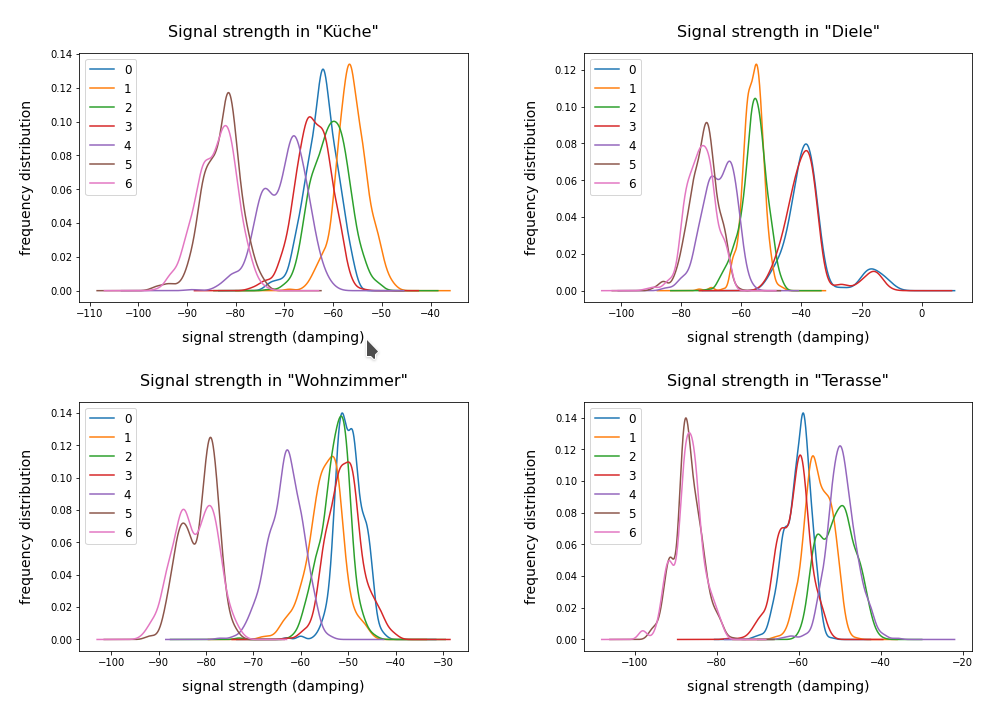# Cluster analysis of the WIFI data – more than four rooms?

The original label-data of the WIFI example imply the existence of four rooms. But can we trust this information? The measurements in the room, which we called „Diele“ in the plots above, indicate a consistent second peak for both the signals 0 and 3. Is this due to an opening into another room?

A simple method to analyze the inner structure of the distribution of data points in a configuration or feature space is a „cluster analysis“. The KMeans algorithm provides such an analysis for an assumed number of clusters.

KMeans is a basic but important ML method which reveals a lot about the data distribution in feature space and indirectly about the complexity of hyperplanes required to separate data according to their labels. Among other things KMeans determines the positions of cluster centers – the so called centroids – by measuring and systematically optimizing distances of samples to assumed centroids. Actually, the sum over all intra-cluster variances, i.e. the summed quadratic distances of the associated samples to their cluster’s centroid, is minimized. The respective quantity is called „inertia“ of the cluster distribution. See e.g. the excellent book of P. Wilmott, Machine learning – an applied mathematics introduction“ on this topic.

A simple method to find out into how many clusters a distribution probably segregates is to look for an elbow in the variation of the inertia with the number of clusters. When we look at the variation of inertia values with the number of potential clusters „k“ for the WIFI example we get the following curve: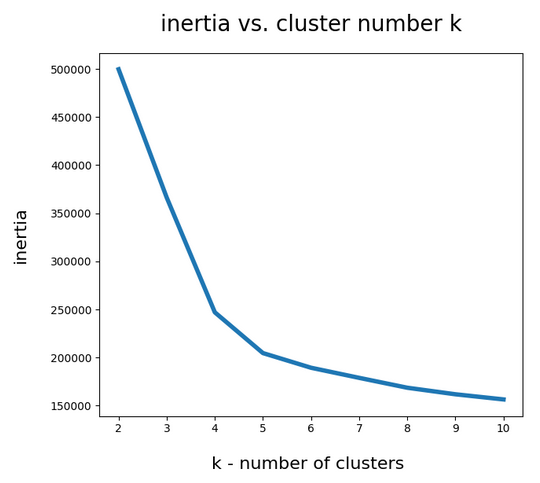This indicates an elbow at k=4,5.

Another method to identify the most probable number of distinct clusters in a multi dimensional data point distribution is the so called „silhouette analysis“. See the book of A. Geron „Hands-On Machine Learning with Scikit-Learn, Keras and Tensorflow“, 2n edition, for a description. For the WIFI example the plots of the silhouette score data support the result of the elbow analysis: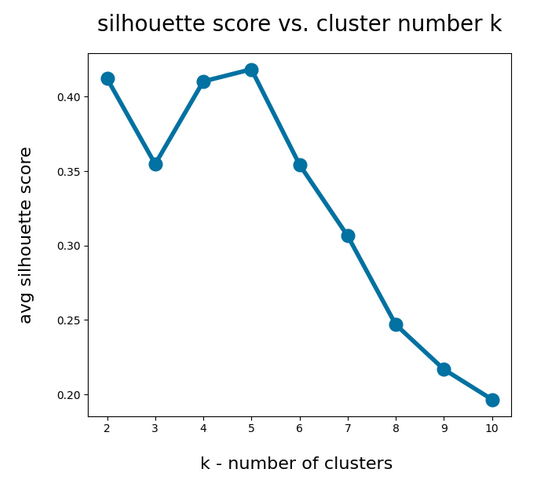The second plot shows ordered silhouette data for k = 3,4,5,6 clusters. Again, we get the most consistent pictures for k=4 and k=5.

So, the data indicate a fragmentation into 4 or 5 clusters. How can we visualize this with respect to the feature space?

# Scatter plots for 2-dim sub-spaces of the feature space

A general problem with the visualization of cluster data for multidimensional data is that we are limited to 2, maximal 3 dimensions. And a projection down to two dimensions may not reflect the real cluster separation in the multidimensional feature space in a realistic way. But sometimes we are lucky.

We shall later see that there are two primary components which dominate the data and signal distributions in the WIFI example. A major question, however, is whether we will also find that only a few original features contribute dominantly to these major components. A PCA analysis does not mean that a „primary component“ only depends on the same number of features!

As I did not know the relation of „primary components“ to features I just plotted the results of „KMeans“ for a variety of 2-dim signal combinations. I used Sklearn’s version of KMeans; due to the very small data ensemble KMeans is applicable without consuming too much CPU time (this will change with MNIST; there we need to invoke MiniBatchKMeans):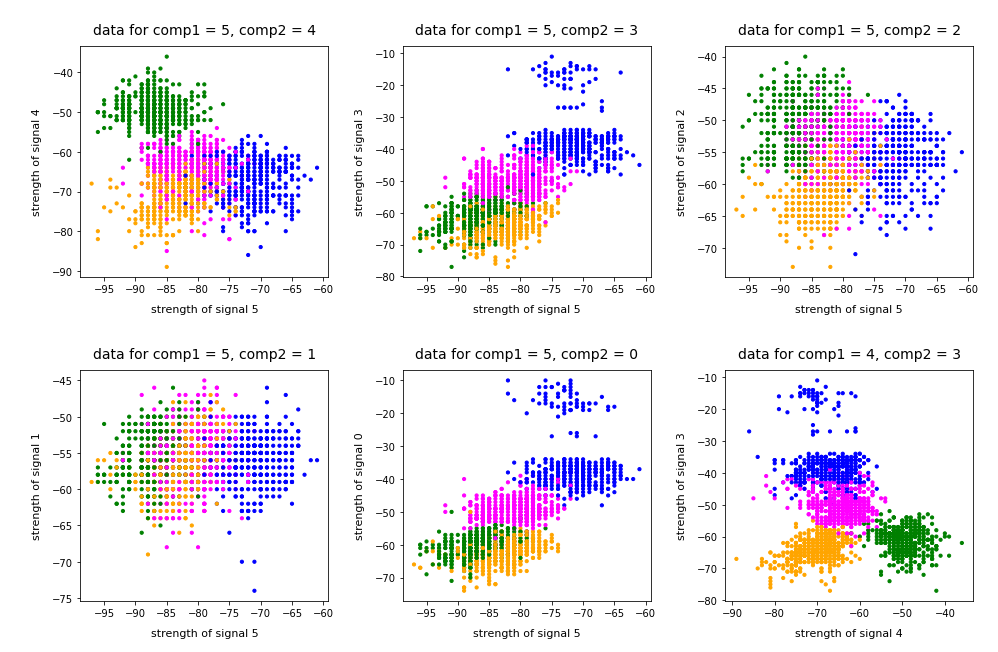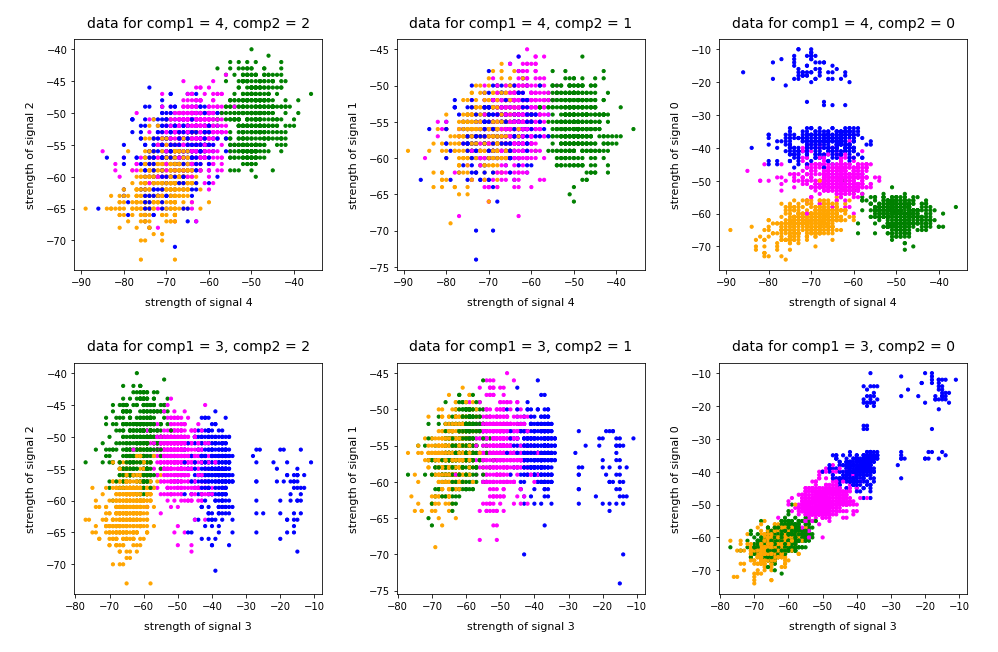Note that the colorization of the data points in all plots was done with respect to the cluster number predicted by KMeans for the samples – and not with respect to their labels.

It is interesting that the projections onto two special feature combinations – namely WLAN-4/WLAN 0 and WLAN-3/WLAN-0 signal – show a very distinct separation of the clusters.

# Four or five clusters ?

The data displayed above depend a bit on the initial distribution of cluster centers as an input into the KMeans algorithm. But for 4 and 5 clusters we get very consistent results. The next plots show the positions of the centroids: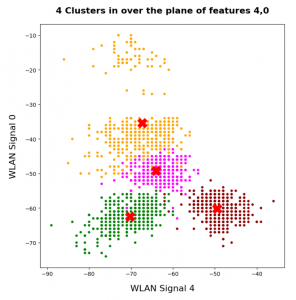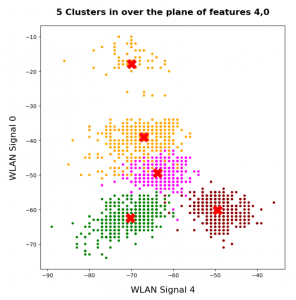This time the colorization was done with respect to the labels. What we see is: Five clusters represent the situation a bit better than only 4 clusters.

When we align this with the rooms: Five „rooms“ may describe the signal variation better than only 4 rooms. The reason for this might be that one of the four rooms has a wall which partially separates different areas from another. We often find this in „entrances“ [German: „Diele“] to houses. Sketches of the rooms in the Linux Magazin article actually show that this is the case. And, of course, such a wall or an opening into another room would have an impact on the damping of the WLAN signals.

# Addendum 19.03.2022: Comparing clusters with groups of labeled data points

An important question which we have not answered yet by the images shown above is the following:

How well do clusters coincide with groups of data points having a specific label?

Note that in general you can not be sure that clusters reflect data points of the same label. Actually, a cluster is only a way to describe a close spatial vicinity of data points in some region of the multidimensional feature space. I.e. some kind of clumping of the data points around some centroids. But spatial vicinity does not necessarily reflect a label: A label border may often separate data points which are very close neighbors. And a cluster may contain a mixture of samples with different labels ….

Well, in the case of the WIFI example the identified 4 to 5 clusters match the groups of data points with different labels quite well. Below I superimposed the sample’s data points with different colors: First I colorized the data points according to their label. On top of the resulting scatter plot I placed the same data points again, but this time with a different and transparent colorization according to their cluster association. In addition I shifted the second data layer a bit to get a better contrast: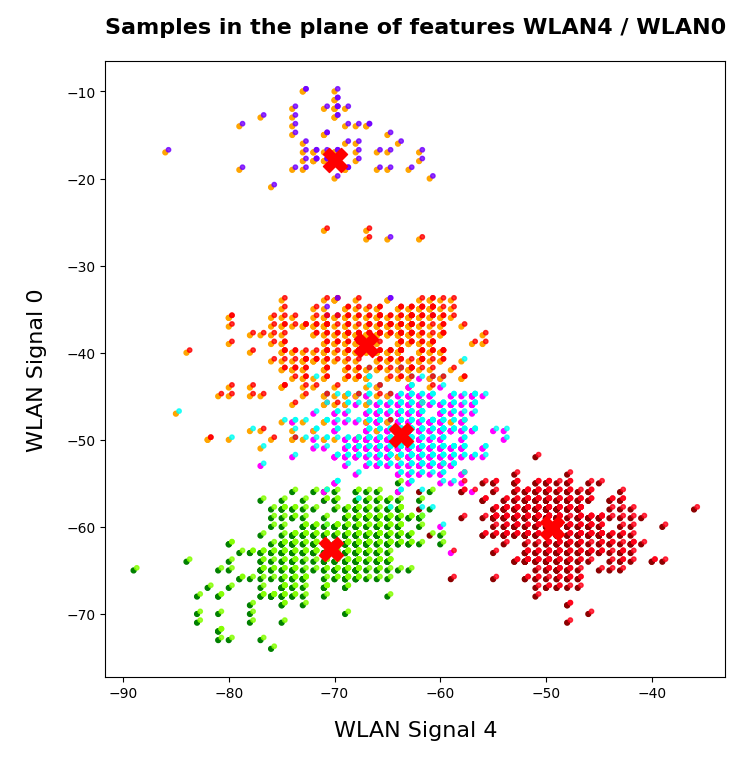You see that the areas are not completely identical, but they overlap quite well. Obviously, I used 5 clusters. Also the fifth cluster fits well into a region characterized by just one label.

# Conclusion

The simple WIFI example shows that a cluster analysis may give you new insights into the structure of ML data sets which a simple classifier algorithm can not provide. In the next article

KMeans as a classifier for the WIFI and MNIST datasets – II – PCA in combination with KMeans for the WIFI-example

we shall link the information contained in the „clusters“ to the results of a PCA analysis of the WIFI example.

Stay tuned …

Ceterum censeo: The most important living fascist which must be denazified is the Putler.

# Deep Dreams of a CNN trained on MNIST data – III – catching dream patterns at smaller length scales

In the first two articles of this series on Convolutional Neural Networks [CNNs] and „Deep Dream“ pictures

I introduced the concept of CNN-based „pattern carving“ and a related algorithm; it consists of a small number of iterations over of a sequence of image manipulation steps (downscaling to a resolution the CNN can handle, detection and amplification of a map triggering pattern by a CNN, upscaling to the original size and normalization). The included pattern detection and amplification algorithm is nothing else than the OIP-algorithm, which I discussed in another article series on CNNs in this blog. The OIP-algorithm is able to create a pattern, which triggers a chosen CNN map optimally, out of a chaotic fluctuation background. The difference is that we apply such an OIP-algorithms now to a structured input image – the basis for a „dream“. The „carving algorithm“ is just a simplifying variation of more advanced Deep Dream algorithms; it can be combined with simple CNNs trained on gray and low-resolution images.

In the last article I provided some code which creates dream like pictures with the help of carving by a CNN trained on MNIST data. Nice, but … In the original theory of „Deep Dreaming“ people from Google and others applied their algorithms to a cascade of so called „octaves„: Octaves represent the structures within an image at different levels of resolution. Unfortunately, at first sight, such an approach seems to be beyond the capabilities of our MNIST CNN, because the latter works on a fixed and very coarse resolution scale of 28×28 pixels, only.

As we cannot work on different scales of resolution directly: Can we handle pattern detection and amplification on different length scales in a different way? Can we somehow extend the carving process to smaller length scales and a possible detection of smaller map-triggering patterns within the input image?
The answer in short is: Yes, we can.
We „just“ have to replace working with „octaves“ by looking at sub-segments of the images – and apply our „carving“ algorithm with up and down-scaling to these sub-segments as well as to the full image during an iteration process. We shall test the effects of such an approach in this article for a single selected sub-area of the input image, before we apply it more thoroughly to different input images in further articles.

# A first step towards Deep Dreams „dreaming“ detail structures of a chosen image …

We again use the image of a bunch of roses, which we worked with in the last articles, as a starting point for the creation of a Deep Dream picture. The easiest way to define sub-areas of our (quadratic) input image is to divide it into 4 (quadratic) adjacent sub-segments or sub-images, each with half of the side length of the original image. We thus get two rows, each with two neighboring sub-areas of the size 280×280 px. Then we could apply the carving algorithm to one selected or to all of the sub-segments. But how do we combine such an approach with an overall treatment of the full image? The answer is that we have to work in parallel on both length-scales involved. We can do this within each cycle of down- and up-scaling according to the following scheme:

• Step 1: Pick the input image IMG at the full working size (here 560 x 560 px) – and reshape it into a tensor suitable for
Tensorflow 2 [TF2] functions.
• Step 2: Down- and upscale the input image „IMG“ (560×560 => 28×28 => 560×560) – with information loss
• Step 3: Calculate the difference between the re-up-scaled image to the original image as a tensor for later detail corrections.
• Step 4: Subdivide IMG into 4 quadrants (each with a size of of 280×280 px). Save the quadrants as sub-images S_IMG_1, …. S_IMG_4 – and create respective tensors.
• Step 5: For all sub-images: Down- and up-scale the sub-images S_IMG_m (280×280 => 28×28 => 280×280) – with information loss.
• Step 6: For all sub-images: Determine the differences between the re-upscaled sub-images and the original sub-images as tensors for later detail corrections.
• Step 7: Loop A [around 4 to 6 iterations]
• Step LA-1: Pick the down-scaled image IMG_d (of size 28×28 px) as the present input image for the CNN and the OIP-analysis
• Step LA-2: Apply the OIP-algorithm for N epochs (20 < N < 40) to the downscaled image IMG_d (28x28)
• Step LA-3: Upscale the resulting changed version of image IMG_d to the original IMG- size by bicubic interpolation => IMG_u
• Step LA-4: Add the (constant) correction tensor for details to IMG_u.
• (Step LA-5: Loop B over Sub-Images)
• Step LB-1: Cut out the area corresponding to sub-image S_IMG_m from the changed full image IMG_u. Use it as Sub_IMG_m during the following steps.
• Step LB-2: Downscale the present sub-image Sub_IMG_m to the size suitable for the CNN (here: 28×28) by bicubic interpolation => Sub_IMG_M_d.
• Step LB-3: Apply the OIP-algorithm for N epochs (20 < N < 40) to the downscaled sub-image (28x28) Sub_IMG_m_d
• Step LB-4: Upscale the changed Sub_IMG_m_d to the original size of Sub_Img_m by bicubic interpolation => Sub_IMG_m_u
• Step LB-5: Add the (constant) correction tensor to the tensor for the upscaled sub-image Sub_IMG_m_u
• Step LB-6: Replace the sub-image region of the upscaled full image IMG_u with the (corrected) sub-image Sub_IMG_m_u
• Step LB-7: Downscale the new full image IMG_u again (to 28×28 => IMG_d) and use the resulting IMG_d for the next iteration of Loop A

The Loop B has been placed in brackets because we are going to apply the suggested technique only to a single one of the 4 sub-image quadrants in this article.

# Code fragments for the sub-image preparation

The following code for a Jupyter cell prepares the quadrants of the original image IMG – here of a bunch of roses. The code is straight forward and easy to understand.

```# ****************************
# Work with sub-Images
# ************************

%matplotlib inline

import PIL
from PIL import Image, ImageOps

fig_size = plt.rcParams["figure.figsize"]
fig_size = 16
fig_size = 20
fig_3 = plt.figure(
3)

# size to work with
# ******************
img_wk_size = 560

# bring the orig img down to (560, 560)
# ***************************************
imgvc = Image.open("rosen_orig_farbe.jpg")
imgvc_wk_size = imgvc.resize((img_wk_size,img_wk_size), resample=PIL.Image.BICUBIC)
# Change to np arrays
ay_picc = np.array(imgvc_wk_size)
print("ay_picc.shape = ", ay_picc.shape)
print("r = ", ay_picc[0,0,0],    " g = ", ay_picc[0,0,1],    " b = " , ay_picc[0,0,2] )
print("r = ", ay_picc[200,0,0],  " g = ", ay_picc[200,0,1],  " b = " , ay_picc[200,0,2] )

# Turn color to gray
#Red * 0.3 + Green * 0.59 + Blue * 0.11
#Red * 0.2126 + Green * 0.7152 + Blue * 0.0722
#Red * 0.299 + Green * 0.587 + Blue * 0.114

ay_picc_g = ( 0.299  * ay_picc[:,:,0] + 0.587  * ay_picc[:,:,1] + 0.114  * ay_picc[:,:,2] )
ay_picc_g = ay_picc_g.astype('float32')
t_picc_g  = ay_picc_g.reshape((1, img_wk_size, img_wk_size, 1))
t_picc_g  = tf.image.per_image_standardization(t_picc_g)

# downsize to (28,28)
t_picc_g_28 = tf.image.resize(t_picc_g, [28,28], method="bicubic", antialias=True)
t_picc_g_28 = tf.image.per_image_standardization(t_picc_g_28)

# get the correction for the full image
t_picc_g_wk_size = tf.image.resize(t_picc_g_28, [img_wk_size,img_wk_size], method="bicubic", antialias=True)
t_picc_g_wk_size = tf.image.per_image_standardization(t_picc_g_wk_size)

t_picc_g_wk_size_corr = t_picc_g - t_picc_g_wk_size
t_picc_g_wk_size_re   = t_picc_g_wk_size + t_picc_g_wk_size_corr

# Display wk_size orig images
ax3_1_1.imshow(imgvc_wk_size)
ax3_1_2.imshow(t_picc_g[0,:,:,0], cmap=plt.cm.gray)
ax3_1_3.imshow(t_picc_g_28[0,:,:,0], cmap=plt.cm.gray)
ax3_1_4.imshow(t_picc_g_wk_size_re[0,:,:,0], cmap=plt.cm.gray)

# Split in 4 sub-images
# ***********************

half_wk_size  = int(img_wk_size / 2)

t_picc_g_1  = t_picc_g[:, 0:half_wk_size, 0:half_wk_size, :]
t_picc_g_2  = t_picc_g[:, 0:half_wk_size, half_wk_size:img_wk_size, :]
t_picc_g_3  = t_picc_g[:, half_wk_size:img_wk_size, 0:half_wk_size, :]
t_picc_g_4  = t_picc_g[:, half_wk_size:img_wk_size, half_wk_size:img_wk_size, :]

# Display wk_size orig images
ax3_2_1.imshow(t_picc_g_1[0,:,:,0], cmap=plt.cm.gray)
ax3_2_2.imshow(t_picc_g_2[0,:,:,0], cmap=plt.cm.gray)
ax3_2_3.imshow(t_picc_g_3[0,:,:,0], cmap=plt.cm.gray)
ax3_2_4.imshow(t_picc_g_4[0,:,:,0], cmap=plt.cm.gray)

# Downscale sub-images
t_picc_g_1_28 = tf.image.resize(t_picc_g_1, [28,28], method="bicubic", antialias=True)
t_picc_g_2_28 = tf.image.resize(t_picc_g_2, [28,28], method="bicubic", antialias=True)
t_picc_g_3_28 = tf.image.resize(t_picc_g_3, [28,28], method="bicubic", antialias=True)
t_picc_g_4_28 = tf.image.resize(t_picc_g_4, [28,28], method="bicubic", antialias=True)

# Display downscales sub-images
ax3_3_1.imshow(t_picc_g_1_28[0,:,:,0], cmap=plt.cm.gray)
ax3_3_2.imshow(t_picc_g_2_28[0,:,:,0], cmap=plt.cm.gray)
ax3_3_3.imshow(t_picc_g_3_28[0,:,:,0], cmap=plt.cm.gray)
ax3_3_4.imshow(t_picc_g_4_28[0,:,:,0], cmap=plt.cm.gray)

# get correction values for upsizing
t_picc_g_1_wk_half = tf.image.resize(t_picc_g_1_28, [half_wk_size,
half_wk_size], method="bicubic", antialias=True)
t_picc_g_1_wk_half = tf.image.per_image_standardization(t_picc_g_1_wk_half)
t_picc_g_2_wk_half = tf.image.resize(t_picc_g_2_28, [half_wk_size,half_wk_size], method="bicubic", antialias=True)
t_picc_g_2_wk_half = tf.image.per_image_standardization(t_picc_g_2_wk_half)
t_picc_g_3_wk_half = tf.image.resize(t_picc_g_3_28, [half_wk_size,half_wk_size], method="bicubic", antialias=True)
t_picc_g_3_wk_half = tf.image.per_image_standardization(t_picc_g_3_wk_half)
t_picc_g_4_wk_half = tf.image.resize(t_picc_g_4_28, [half_wk_size,half_wk_size], method="bicubic", antialias=True)
t_picc_g_4_wk_half = tf.image.per_image_standardization(t_picc_g_4_wk_half)

t_picc_g_1_corr =  t_picc_g_1 - t_picc_g_1_wk_half
t_picc_g_2_corr =  t_picc_g_2 - t_picc_g_2_wk_half
t_picc_g_3_corr =  t_picc_g_3 - t_picc_g_3_wk_half
t_picc_g_4_corr =  t_picc_g_4 - t_picc_g_4_wk_half

t_picc_g_1_re   = t_picc_g_1_wk_half + t_picc_g_1_corr
t_picc_g_2_re   = t_picc_g_2_wk_half + t_picc_g_2_corr
t_picc_g_3_re   = t_picc_g_3_wk_half + t_picc_g_3_corr
t_picc_g_4_re   = t_picc_g_4_wk_half + t_picc_g_4_corr

print(t_picc_g_1_re.shape)

# Display downscales sub-images
ax3_4_1.imshow(t_picc_g_1_re[0,:,:,0], cmap=plt.cm.gray)
ax3_4_2.imshow(t_picc_g_2_re[0,:,:,0], cmap=plt.cm.gray)
ax3_4_3.imshow(t_picc_g_3_re[0,:,:,0], cmap=plt.cm.gray)
ax3_4_4.imshow(t_picc_g_4_re[0,:,:,0], cmap=plt.cm.gray)

ay_img_comp = np.zeros((img_wk_size,img_wk_size))
ay_t_comp   = ay_img_comp.reshape((1,img_wk_size, img_wk_size,1))
t_pic_comp  = ay_t_comp
t_pic_comp[0, 0:half_wk_size, 0:half_wk_size, 0]                       = t_picc_g_1_re[0, :, :, 0]
t_pic_comp[0, 0:half_wk_size, half_wk_size:img_wk_size, 0]             = t_picc_g_2_re[0, :, :, 0]
t_pic_comp[0, half_wk_size:img_wk_size, 0:half_wk_size, 0]             = t_picc_g_3_re[0, :, :, 0]
t_pic_comp[0, half_wk_size:img_wk_size, half_wk_size:img_wk_size, 0]   = t_picc_g_4_re[0, :, :, 0]

ax3_5_1.imshow(t_picc_g[0,:,:,0], cmap=plt.cm.gray)
ax3_5_2.imshow(t_pic_comp[0,:,:,0], cmap=plt.cm.gray)

```

Note the computation of the correction tensors; we prove their effectiveness below by displaying respective results for the full image, cut out and re-upscaled, corrected quadrants and replaced areas of the original image.

The results in the prepared image frames look like:Good!

# Some code for the carving algorithm – applied to the full image AND a single selected sub-image

Now, for a first test, we concentrate on the sub-image in the lower-right corner – and apply the above algorithm. A corresponding Jupyter cell code is given below:

```# *************************************************************************
# OIP analysis on sub-image tiles (to be used after the previous cell)
# **************************************************************************
# Note: To be applied after previous cell !!!
# ******

#interactive plotting - will be used in a future version when we deal with "octaves"
#%matplotlib notebook
#plt.ion()

%matplotlib inline

# preparation of figure frames
# ~~~~~~~~~~~~~~~~~~~~~~~~~~~~~
fig_size = plt.rcParams["figure.figsize"]
fig_size = 12
fig_size = 12
fig_4 = plt.figure(4)

ax4_2_

fig_size = plt.rcParams["figure.figsize"]
fig_size = 12
fig_size = 6
fig_5 = plt.figure(5)
li_axa5 = [axa5_1, axa5_2, axa5_3, axa5_4, axa5_5, axa5_6, axa5_7, axa5_8]

# Some general OIP run parameters
# ~~~~~~~~~~~~~~~~~~~~~~~~~~~~~~~~~
n_epochs  = 30          # should be divisible by 5
n_steps   = 6           # number of intermediate reports
epsilon   = 0.01        # step size for gradient correction
conv_criterion = 2.e-4  # criterion for a potential stop of optimization

# image width parameters
# ~~~~~~~~~~~~~~~~~~~~~
img_wk_size   = 560    # must be identical to the last cell
half_wk_size  = int(img_wk_size / 2)

# min / max values of the input image
#~~~~~~~~~~~~~~~~~~~~~~~~~~~~~~~~~~~~
# Note : to be used for contrast enhancement
min1 = tf.reduce_min(t_picc_g)
max1 = tf.reduce_max(t_picc_g)
# parameters to deal with the spectral distribution of gray values
spect_dist = min(abs(min1), abs(max1))
spect_fact   = 0.85
height_fact  = 1.1

# Set the gray downscaled input image as a startng point for the iteration loop
# ~~~~~~~~~~~~~----------~~~~--------------~~~~~~~~~~~~~~~~~~~~~~~~~~~~~~~~~~~~~~~~
MyOIP._initial_inp_img_data = t_picc_g_28

# ************
# Main Loop
# ***********

num_main_iterate = 4
# map_index_main = -1       # take a cost function for a full layer
map_index_main = 56         # map-index to be used for the OIP analysis of the full image
map_index_sub  = 56         # map-index to be used for the OIP analysis of the sub-images

for j in range(num_main_iterate):

# ******************************************************
# deal with the full (downscaled) input image
# ******************************************************

# Apply OIP-algorithm to the whole downscaled image
# ~~~~~~~~~~~~~~~~~~~-----------~~~~~~~~~~~~~~~~~~~~~~~
map_index_main_oip = map_index_main   # map-index we are interested in

# Perform the OIP analysis
MyOIP._derive_OIP(map_index = map_index_oip,
n_epochs = n_epochs, n_steps = n_steps,
epsilon = epsilon , conv_criterion = conv_criterion,
b_stop_with_convergence=False,
b_print=False,
li_axa = li_axa5,
ax1_1 = ax4_1_1, ax1_2 = ax4_1_2)

# display the modified downscaled image  (without and with contrast)
# ~~~~~~~~~~~~~~~~~~~~~~~~~~~~~~~~~~~~~~
t_oip_g_28  = MyOIP._inp_img_data
ay_oip_g_28 = t_oip_g_28[0,:,:,0].numpy()
ay_oip_g_28_cont = MyOIP._transform_tensor_to_img(T_img=t_oip_g_28[0,:,:,0], centre_move=0.33, fact=1.0)
ax4_1_3.imshow(ay_oip_g_28_cont, cmap=plt.cm.gray)
ax4_1_4.imshow(t_picc_g_28[0, :, :, 0], cmap=plt.cm.gray)

# rescale to 560 and re-add details via the correction tensor
# ~~~~~~~~~~~~~~~~~~~~~~~~~~~~~~~~~~~~~~~~~~~~~~~~~~~~~~~~~~~~~
t_oip_g_wk_size        = tf.image.resize(t_oip_g_28, [img_wk_size,img_wk_size],
method="bicubic", antialias=True)
t_oip_g_wk_size_re     = t_oip_g_wk_size + t_picc_g_wk_size_corr

# standardize to get an intermediate image
# ~~~~~~~~~~~~~~~~~~~~~~~~~~~~~~~~~~~~~~~~~~~~
n    # t_oip_g_loop           = t_oip_g_wk_size_re.numpy()
# t_oip_g_wk_size_re_std = tf.image.per_image_standardization(t_oip_g_wk_size_re)
t_oip_g_wk_size_re_std = tf.image.per_image_standardization(t_oip_g_wk_size_re)
t_oip_g_loop           = t_oip_g_wk_size_re_std.numpy()

# contrast required as the reduction of irrelvant pixels has smoothed out the gray scale beneath
# ~~~~~~~~~~~~~~~~~
# the added details at pattern areas = high level of whitened socket + relative small detail variation
t_oip_g_wk_size_re_std_plt = tf.clip_by_value(height_fact*t_oip_g_wk_size_re_std, -spect_dist*spect_fact, spect_dist*spect_fact)
ax4_2_1.imshow(t_oip_g_wk_size[0,:,:,0], cmap=plt.cm.gray)
ax4_2_2.imshow(t_oip_g_wk_size_re_std[0,:,:,0], cmap=plt.cm.gray)
ax4_2_3.imshow(t_oip_g_wk_size_re_std_plt[0,:,:,0], cmap=plt.cm.gray)
ax4_2_4.imshow(t_picc_g[0,:,:,0], cmap=plt.cm.gray)

# *************************************************************
# deal with a chosen sub-image - in this version: "t_oip_4_g"
# **************************************************************

# Cut out and downscale a sub-image region
# ~~~~~~~~~~~~~~~~~~~~~~~~~~~~~~~~~~~~~~~~~
t_oip_4_g     = t_oip_g_loop[:, half_wk_size:img_wk_size, half_wk_size:img_wk_size, :]
t_oip_4_g_28  = tf.image.resize(t_oip_4_g, [28,28], method="bicubic", antialias=True)

# use the downscaled sub-image as an input image to the OIP-algorithm
# ~~~~~~~~~~~~~~~~~~~~~~~~~~~~~~~~~~~~~~~~~~~~~~~~~~~~~~~~~~~~~~~~~~~~
MyOIP._initial_inp_img_data = t_oip_4_g_28

# Perform the OIP analysis on the sub-image
# ~~~~~~~~~~~~~~~~~~~~~~~~~~~~~~~~~~~~~~~~~~~
map_index_sub_oip  = map_index_sub   # we may vary this in later versions

MyOIP._derive_OIP(map_index = map_index_sub_oip,
n_epochs = n_epochs, n_steps = n_steps,
epsilon = epsilon , conv_criterion = conv_criterion,
b_stop_with_convergence=False,
b_print=False,
li_axa = li_axa5,
ax1_1 = ax4_3_1, ax1_2 = ax4_3_2)

# display the modified sub-image without and with contrast
# ~~~~~~~~~~~~~~~~~~~~~~~~~~~~~~~~~~~~~~~~~~~~~~~~~~~~~~~~~
t_oip_4_g_28  = MyOIP._inp_img_data
ay_oip_4_g_28 = t_oip_4_g_28[0,:,:,0].numpy()
ay_oip_4_g_28_cont = MyOIP._transform_tensor_to_img(T_img=t_oip_4_g_28[0,:,:,0], centre_move=0.33, fact=1.0)
ax4_3_3.imshow(ay_oip_4_g_28_cont, cmap=plt.cm.gray)
ax4_3_4.imshow(t_oip_4_g[0, :, :, 0], cmap=plt.cm.gray)

# upscaling of the present sub-image
# ~~~~~~~~~~~~~~~~~~~~~~~~~~~~~~~~~~~
t_pic_4_g_wk_half = tf.image.resize(t_oip_4_g_28, [half_wk_size,half_wk_size], method="bicubic", antialias=True)

# add the detail correction to the manipulated upscaled sub-image
# ~~~~~~~~~~~~~~~~~~~~~~~~~~~~~~~~~~~~~~~~~~~~~~~~~~~~~~~~~~~~~~~~
t_pic_4_g_wk_half = t_pic_4_g_wk_half + t_picc_g_4_corr
#t_pic_4_g_wk_half = tf.image.per_image_standardization(t_pic_4_g_wk_half)
ax4_4_1.imshow(t_pic_4_g_wk_half[0, :, :, 0], cmap=plt.cm.gray)
ax4_4_2.imshow(t_oip_4_g[0, :, :, 0], cmap=plt.cm.gray)

# Overwrite the related region in the full image with the new sub-image
# ~~~~~~~~~~~~~~~~~~~~~~~~~~~~~~~~~~~~~~~~~~~~~~~~~~~~~~~~~~~~~~~~~~~~~
t_oip_g_loop[0, half_wk_size:img_wk_size, half_wk_size:img_wk_size, 0]  = t_pic_4_g_wk_half[0, :, :, 0]
t_oip_g_loop_std = tf.image.per_image_standardization(t_oip_g_loop)

#ax4_4_3.imshow(t_oip_g_loop[0, :, :, 0], cmap=plt.cm.gray)
ax4_4_3.imshow(t_oip_g_loop_std[0, :, :, 0], cmap=plt.cm.gray)
ay_oip_g_loop_std_plt = tf.clip_by_value(height_fact*t_oip_g_loop_std, -spect_dist*spect_fact, spect_dist*spect_fact)
ax4_4_4.
imshow(ay_oip_g_loop_std_plt[0, :, :, 0], cmap=plt.cm.gray)

# Downscale the resulting new full image and feed it into into the next iteration of the main loop
# ~~~~~~~~~~~~~~~~~~~~~~~~~~~~~~~~~~~~~~~~~~~~~~~~~~~~~~~~~~~~~~~~~~~~~~~~~~~~~~~~~~~~~~~~~~~~~~~~~
MyOIP._initial_inp_img_data = tf.image.resize(t_oip_g_loop_std, [28,28], method="bicubic", antialias=True)

```

The result of these operations after 4 iterations is displayed in the following image:We recognize again the worm-like shape resulting for map 56, which we have seen in the last article, already. (By the way: Our CNN’s map 56 originally is strongly triggered for the shapes of handwritten 9-digits and plays a significant role in classifying respective images correctly). But there is a new feature which appeared in the lower right part of the image – a kind of wheel or two closely neighbored 9-like shapes.

The following code compares the final result with the original image:

```fig_size = plt.rcParams["figure.figsize"]
fig_size = 12
fig_size = 12
fig_7X = plt.figure(7)

ay_pic_full_cont = MyOIP._transform_tensor_to_img(T_img=t_oip_g_loop_std[0, :, :, 0], centre_move=0.46, fact=0.8)

ax1_7X_1.imshow(imgvc_wk_size)
ax1_7X_2.imshow(t_picc_g[0,:,:,0], cmap=plt.cm.gray)
ax1_7X_3.imshow(ay_oip_g_loop_std_plt[0, :, :, 0], cmap=plt.cm.gray)
ax1_7X_4.imshow(ay_pic_full_cont, cmap=plt.cm.gray)
```

Giving: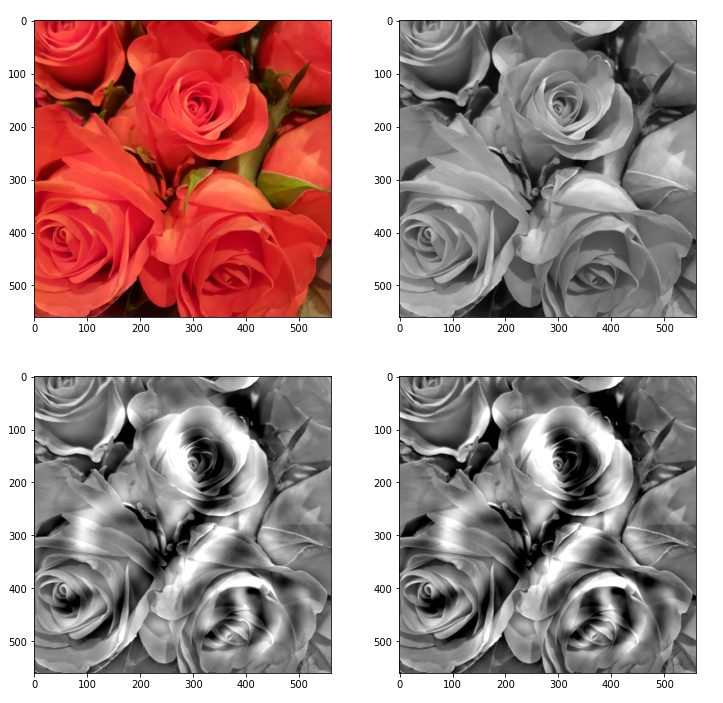Those who do not like the relative strong contrast may do their own experiments with other parameters for contrast enhancement.

You see: Simplifying prejudices about the world, which get or got manifested in inflexible neural networks (or brains ?), may lead to strange, if not bad dreams. May also some politicians learn from this … hmmm, just joking, as this requires both an openness and capacity for learning … can’t await Jan, 21st …

# Conclusion

Our „carving algorithm“, which corresponds to an amplification of traces of map-related patterns that a CNN detects in an input image, can also be used for sub-image-areas and their pixel information. We can therefore use even very limited CNNs trained on low resolution MNIST images to create a „Deep MNIST Dream“ out of a chosen arbitrary input image. At least in principle.

Addendum 06.01.2021: There is, however, a pitfall – our CNN (for MNIST or other image samples) may have been trained and work on standardized image/pixel data, only. But even if the overall input image may have been standardized before operating on it in the sense of the algorithm discussed in this article, we should take into account that a sub-image area may contain pixel data which are far from a standardized distribution. So, our corrections for details may require more than just the calculation of a difference term. We may have to reverse intermediate standardization steps, too. We shall take care of this in forthcoming code changes.

In the next article we are going to extend our algorithm to multiple cascaded sub-areas of our image – and we vary the maps used for different sub-areas at the same time.